Next: Gradient Up: Vector Algebra and Vector Previous: Vector Line Integrals

Volume Integrals

A volume integral takes the form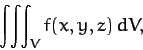(1341)

where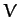is some volume, and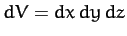is a small volume element. The volume element is sometimes written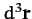, or even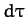.

As an example of a volume integral, let us evaluate the center of gravity of a solid pyramid. Suppose that the pyramid has a square base of side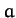, a height, and is composed of material of uniform density. Let the centroid of the base lie at the origin, and let the apex lie at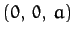. By symmetry, the center of mass lies on the line joining the centroid to the apex. In fact, the height of the center of mass is given by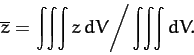(1342)

The bottom integral is just the volume of the pyramid, and can be written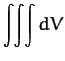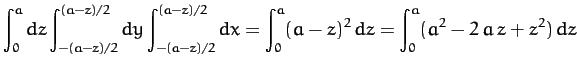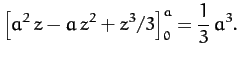(1343)

Here, we have evaluated the-integral last because the limits of the- and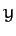- integrals are-dependent. The top integral takes the form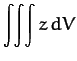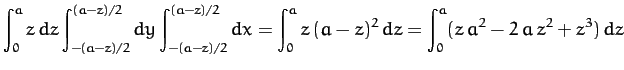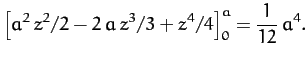(1344)

Thus,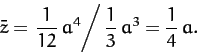(1345)

In other words, the center of mass of a pyramid lies one quarter of the way between the centroid of the base and the apex.Next: Gradient Up: Vector Algebra and Vector Previous: Vector Line Integrals
Richard Fitzpatrick 2011-03-31